# MATLBA中最小二乘支持向量机原理+实例分析

1、最小二乘支持向量机LSSVM基本原理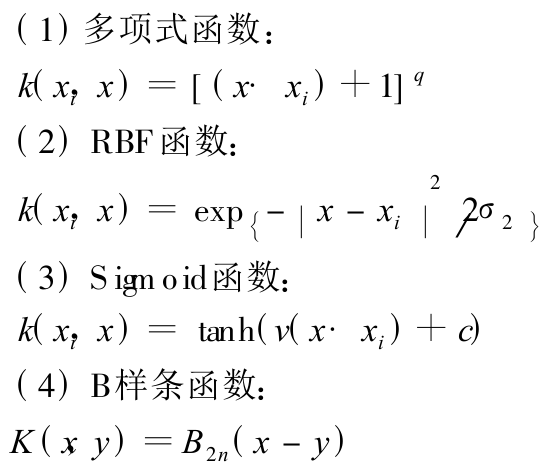2、LSSVM工具箱的使用方法

2.1 最小二乘支持向量机Matlab工具箱下载链接：https://www.esat.kuleuven.be/sista/lssvmlab/（毫无疑问下载最新版本）

2.2 将LS-SVM文件添加到matlan使用路径中，便可直接使用。

1 导入训练数据：load 读取mat文件和ASCII文件；xlsread读取.xls文件；csvread读取.csv文件。

2 数据预处理：效果是加快训练速度。

标准化处理(把每组数据都化为均值为 0, 方差为 1的一组数据, 所涉及到的函数有 prestd,poatstd, trastd)

主成分分析 (进行正交处理, 减少输入数据的维数, 所涉及到的函数有 prepca, trapca)

3  LS-SVM lab用于函数回归主要用到 3个函数, trainlssvm函数用来训练建立模型, simlssvm函数用于预估模型, plotlssvm函数是 LS-SVM lab工具箱的专用绘图函数。

4 参数说明：

A =csvread(′traindata. csv′);

Ptrain0=A(:, [ 1:13] );Ttrain0=A(:, [ 14:16);
[ Ptrain, meanptrain, stdptrain] = prestd(Ptrain0′);
[ Ttrain, meant , stdt] = prestd(T train0′);
Prestd()是数据归一化函数, 其中 meanptrain是未归一化数据之前的向量平均值 stdptrain是未归一化数据之前的向量标准差。

gam =10;sig2=0. 5;type=′function estimation′;
LS-SVM 要求调的参数就两个。 gam 和 sig2是最小二乘支持向量机的参数, 其中 gam 是正则化参数, 决定了适应误差的最小化和平滑程度, sig2是 RBF 函数的参数。 在工具箱中有一个函数 gridsearch可以在一定的范围内用来寻找最优的参数范围。 type有两种类型, 一种是 classfication, 用于分类, 一种是 function estimation, 用于函数回归。

[ alpha, b] =trainlssvm({Ptrain′, Ttrain′, type, gam, sig2,′RBF_kernel′, ′preprocess′});

alpha是支持向量, b是阈值. 。 preprocess是表明数据已经进行归一化, 也可以是′original ′, 表明数据没有进行归一化, 缺省时是′preprocess′。

plotlssvm ({P, T, type, gam, sig2, ′RBF _ kernel ′,′preprocess′}, {alpha, b})plotlssvm函数是 LS-SVM 工具箱特有的绘图函数, 和 plot函数原理相近。

simlssvm函数也是 LS-SVM 工具箱的重要函数, 其中的参数如上述所示, 原理类似于神经网络工具箱中的 sim 函数。

3、示例程序：回归问题

close all;clear,clc;
X = (-3:0.2:3)';
Y = sinc(X)+0.1.*randn(length(X),1);
plot(X,Y,'*')
gam = 10;sig2 = 0.3;type = 'function estimation';
% 建模
[alpha,b] = trainlssvm({X,Y,type,gam,sig2,'RBF_kernel','preprocess'});      % 数据归一化处理
% 画图
figure; plotlssvm({X,Y,type,gam,sig2,'RBF_kernel','preprocess'},{alpha,b});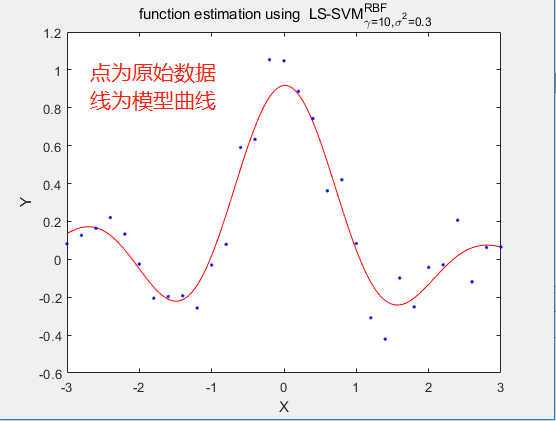close all;clear,clc;
X = (-3:0.2:3)';
Y = sinc(X)+0.1.*randn(length(X),1);
gam = 10;sig2 = 0.3;type = 'function estimation';
% 建模
[alpha,b] = trainlssvm({X,Y,type,gam,sig2,'RBF_kernel','preprocess'});
Xt = 3.*randn(10,1);
Xt = (min(X):.1:max(X))';
Yt = simlssvm({X,Y,type,gam,sig2,'RBF_kernel','preprocess'},{alpha,b},Xt);
% 真实数据
Ytt = sinc(Xt)+0.1.*randn(length(Xt),1);
plot(Xt,Yt,'*r')
hold on
plot(Xt,Ytt,'*b')
figure; plotlssvm({X,Y,type,gam,sig2,'RBF_kernel','preprocess'},{alpha,b});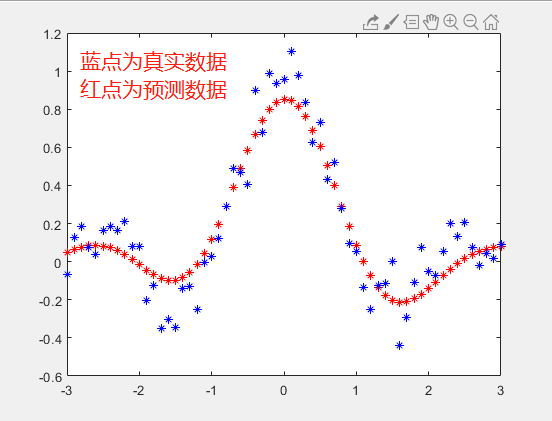close all;clear;clc;
X = 2.*rand(30,2)-1;
Y = sign(sin(X(:,1))+X(:,2));
% 参数设置
gam = 10;sig2 = 0.2;type = 'classification';
% 建模和图形化显示
[alpha,b] = trainlssvm({X,Y,type,gam,sig2,'RBF_kernel','preprocess'});
figure; plotlssvm({X,Y,type,gam,sig2,'RBF_kernel','preprocess'},{alpha,b});
% 利用训练模型进行预测
Xt = 2.*rand(10,2)-1;
disp(' >> Ytest = simlssvm({X,Y,type,gam,sig2,''RBF_kernel'',''preprocess''},{alpha,b},Xt);');
Ytest = simlssvm({X,Y,type,gam,sig2,'RBF_kernel','preprocess'},{alpha,b},Xt);
% 利用公式计算真实值，并与模型预测结果进行对比
YY = sign(sin(Xt(:,1))+Xt(:,2));
figure
subplot(2,1,1)
plot(Xt,Ytest,'*'); title('模型预测结果','fontsize',13);
subplot(2,1,2)
plot(Xt,YY,'*'); title('公式计算结果','fontsize',13);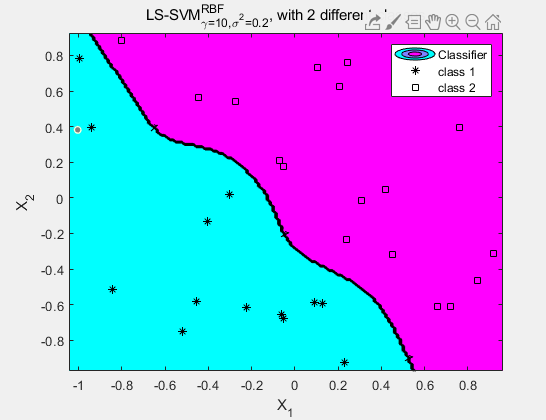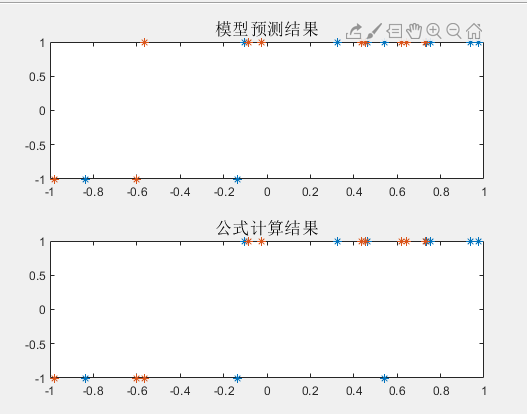09-27
06-293万+
03-2849
11-162万+
02-141万+
11-182万+
11-30
03-27304
07-20807
10-054848
08-249195
04-131万+
08-041187
©️2020 CSDN 皮肤主题: 大白 设计师:CSDN官方博客